# Online Mechanical Engineering Test - Hydraulics and Fluid Mechanics TestLoading Test...

Instruction:

• This is a FREE online test. DO NOT pay money to anyone to attend this test.
• Total number of questions : 20.
• Time alloted : 30 minutes.
• Each question carry 1 mark, no negative marks.
• DO NOT refresh the page.
• All the best :-).

1.

The coefficient of discharge for an external mouthpiece is

A.
 0.375B.
 0.5C.
 0.707D.
 0.8552.

A compound pipe of diameter d1, d2 and d3 having lengths l1, l2 and l3 is to be replaced by an equivalent pipe of uniform diameter d and of the same length (l) as that of the compound pipe. The size of the equivalent pipe is given by

A.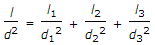B.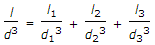C.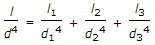D.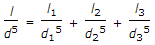3.

The volume per unit mass of a liquid is called specific volume.

A.
 YesB.
 No4.

The loss of head at entrance in a pipe is (where v = Velocity of liquid in the pipe)

A.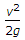B.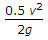C.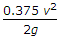D.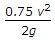5.

Select the correct statement

A.
 Weber's number is the ratio of inertia force to elastic force.B.
 Weber's number is the ratio of gravity force to surface tension force.C.
 Weber's number is the ratio of viscous force to pressure force.D.
 Weber's number is the ratio of inertia force to surface tension force.6.

The hydraulic mean depth or the hydraulic radius is the ratio of

A.
 area of flow and wetted perimeterB.
 wetted perimeter and diameter of pipeC.
 velocity of flow and area of flowD.
 none of these7.

The power absorbed (in watts) in overcoming the viscous resistance of a footstep bearing is

A.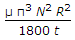B.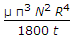C.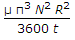D.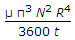8.

The hydraulic mean depth for a circular pipe of diameter (d) is

A.
 d/6B.
 d/4C.
 d/2D.
 d9.

A notch is used to measure __________ of liquids.

A.
 pressureB.
 dischargeC.
 velocityD.
 volume10.

A flow is called sonic, if the Mach number is unity.

A.
 AgreeB.
 Disagree11.

The magnitude of water hammer depends upon the

A.
 elastic properties of the pipe materialB.
 elastic properties of the liquid flowing through the pipeC.
 speed at which the valve is closedD.
 all of the above12.

The most efficient section of a channel is

A.
 triangularB.
 rectangularC.
 squareD.
 trapezoidal13.

The depth of centre of pressure (h) for a vertically immersed surface from the liquid surface is given by (where IG = Moment of inertia of the immersed surface about horizontal axis through its centre of gravity, A = Area of immersed surface, and x = Depth of centre of gravity of the immersed surface from the liquid surface)

A.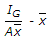B.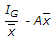C.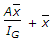D.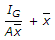14.

The velocity at which the turbulent flow starts is known as higher critical velocity.

A.
 YesB.
 No15.

The rise of liquid along the walls of a revolving cylinder about the initial level is __________ the depression of the liquid at the axis of rotation.

A.
 same asB.
 less thanC.
 more than16.

According to Manning's formula, the discharge through an open channel is (where M = Manning's constant)

A.
 A x M x m1/2 x i2/3B.
 A x M x m2/3 x i1/2C.
 A1/2 x M2/3 x m x iD.
 A2/3 x M1/3 x m x i17.

Newton's law of viscosity is a relationship between

A.
 pressure, velocity and temperatureB.
 shear stress and rate of shear strainC.
 shear stress and velocityD.
 rate of shear strain and temperature18.

A body floating in a liquid is said to be not in equilibrium if its metacentre lies below its centre of gravity.

A.
 AgreeB.
 Disagree19.

When the flow in an open channel is gradually varied, the flow is said to be

A.
 steady uniform flowB.
 steady non-uniform flowC.
 unsteady uniform flowD.
 unsteady non-uniform flow20.

A flow through an expanding tube at constant rate is called

A.
 steady uniform flowB.
 steady non-uniform flowC.
 unsteady uniform flowD.
 unsteady non-uniform flow